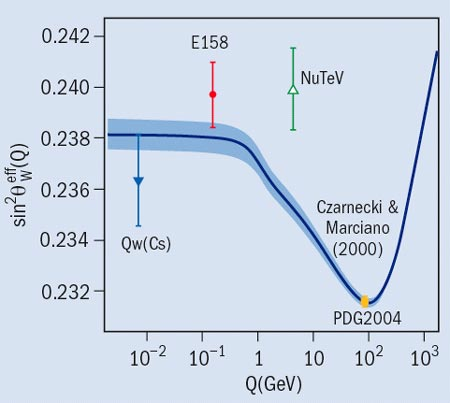# Variation in weak force found by E158

17 July 2005

The E158 experiment at the Stanford Linear Accelerator Center has made a landmark observation: the strength of the weak force acting on two electrons lessens when the electrons are far apart. The results will be published in Physical Review Letters.Predicted variation of sin2θweff as a function of momentum transfer Q (solid line) and its estimated theoretical uncertainty (shaded). Results of previous low-energy experiments are shown with the Particle Data Group value (PDG2004) at the scale of the Z mass.

Because there is an asymmetry in how the weak force acts, there is a difference between how often left- and right-handed electrons scatter via a Z particle (the neutral carrier of the weak force). Two years ago, the team made the first observation of this parity-violation effect in electron-electron interactions.

For the new results, E185 used its improved precision asymmetry measurement to calculate the long-distance (low momentum transfer, Q) weak charge of the electron, which determines the strength of the weak force between two electrons. The result is the world’s best determination of the weak mixing angle at low energy: sin2θweff = 0.2397 ± 0.0010 (stat.) ± 0.0008 (syst.), evaluated at Q2 = 0.026 GeV2.

Previous experiments at SLAC and CERN measured the electron’s weak charge at high momentum transfer (short distances). E158’s long-distance measurement observes this weak charge to be half the size of the charge at short distances. Comparing the short-distance measurements with the long-distance results demonstrates (with 6σ significance) the variation of the strength of the weak force with distance, and confirms an important aspect of Standard Model theory. Using the result for sin2θweff, E158 finds the electron’s weak charge to be -0.041 ± 0.006 – half the value expected if there were no variation.

E158 was also sensitive to indirect signals from hypothetical Z’ particles, suggesting they are at least 10 times the mass of the Z.